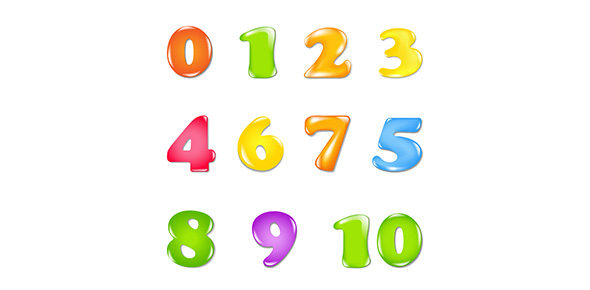# Classifying Numbers

5 Questions | Total Attempts: 1781SettingsThis is a quiz on classifying numbers

Related Topics
• 1.
Which of the following is an integer
• A.

2

• B.

.21

• C.

1/4

• D.

1/25

• 2.
The number zero can be classified as :
• A.

Counting number

• B.

Irrational number

• C.

Whole number

• D.

Imaginary number

• 3.
Which of the following best classifies the number 3
• A.

Whole number

• B.

Counting number

• C.

Real Number

• D.

All the above

• 4.
1/9 is a
• A.

Rational Number

• B.

Irrational Number

• C.

Whole Number

• D.

Integer

• 5.
Pi is which of the following
• A.

Real Number

• B.

Imaginary Number

• C.

Irrational Number

• D.

Integer